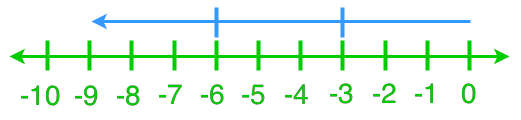# Dividing integers#### Everything You Need in One Place

Homework problems? Exam preparation? Trying to grasp a concept or just brushing up the basics? Our extensive help & practice library have got you covered.#### Learn and Practice With Ease

Our proven video lessons ease you through problems quickly, and you get tonnes of friendly practice on questions that trip students up on tests and finals.#### Instant and Unlimited Help

Our personalized learning platform enables you to instantly find the exact walkthrough to your specific type of question. Activate unlimited help now!##### Intros
###### Lessons
1. Introduction to division statements with non-integer quotients
##### Examples
###### Lessons
1. Understanding Division Using a Number Line
What are the two division statements that each diagram represents?

1.2.2. Solving Division Statements Using a Number Line
Use a number line to find each quotient.
1. (+10)÷(+5)
2. (+8)÷(-2)
3. (-12)÷(+4)
4. (-9)÷(-3)
3. Division Statements With Integer Quotients
Solve.
1. (+36)÷(-6)
2. (-45)÷(+15)
3. (-28)÷(-4)
4. 0÷(-16)
4. Division Statements With Non-Integer Quotients
Solve.
1. (-36)÷(+8)
2. (-100)÷(-3)
3. (+47)÷(+6)
4. (+34)÷(-7)
5. Word Problems of Dividing Integers
Katie participated in a track competition. If her average sprinting speed is 8 m/s, how long does it take for her to finish an 100 m sprint?
1. Peter finished a 45 km long cycling trail. He first cycled for 3 hours and then, he took a break. Afterwards Peter cycled for another 2 hours to finish the trail. What was his cycling rate per hour? Were there any assumptions?
##### Practice
###### Topic Notes
In this section, we will keep working on dividing integers. We will learn how to read and represent division statements on a number line. We will then practice more integer divisions with actual numbers and word problems.

In this lesson, we will learn:

• Understanding Division Using a Number Line
• Solving Division Statements Using a Number Line
• Division Statements With Integer Quotients
• Division Statements With Non-Integer Quotients
• Word Problems of Dividing Integers

Notes:
• Recurring/ repeating decimals: decimal numbers whose digits repeat forever.
• For the recurring decimals, we put either a bar or dots above the recurring parts.